# User Guides - Functions List

## By Alexander Yee

(Last updated: March 24, 2019)

Back To:

This is the function reference for the Custom Formula feature in y-cruncher.

 Support Basic Arithmetic Functions Built-in Constants Binary Splitting Series Function Templates Integer LinearCombination Invsqrt(x) GoldenRatio SeriesHyperdescent ArcTan(1/x) for integer x - Taylor Series Scope Multiply Sqrt(x) E SeriesHypergeometric ArcTan(1/x) for integer x - Euler Series Divide AGM(1,x) and AGM(a,b) Pi SeriesBinaryBBP ArcTanh(x) for real x Shift Log(x) Zeta3 ArcCoth(x) for real x Integer Power Log(x) with precomputed constants Catalan ArcSinh(x) for real x ArcCoth(x) for integer x Lemniscate ArcCosh(x) for real x ArcSinlemn(x) for rational x EulerGamma

It should be noted that the functionality here isn't exactly meant to be user-friendly or intuitive. Instead, these functions should be seen as portals to y-cruncher's internal functionality with little to no overhead. Therefore, writing a custom formula is akin to writing assembly language where the "instructions" are calls to y-cruncher's native internal functionality.

• The functionality here exposes the inverse square root since it is faster to compute than the square root itself. The square root function itself is just a convenience wrapper that calls the inverse square root and then a large multiply. There is no native square root function.

• Log(x) for integer x restricts its inputs to half-word integers due to that being the limitation of the internal Machin-like formula implementation. If you want to input a larger x, you can easily work-around this restriction using the Integer function to convert it into a large number. But doing so will call the much slower AGM-based algorithm.

• The Power function is an overloaded function that can map to one or more of several different internal functions depending on the value of the exponent.

• LinearCombination is a non-native function. It maps to multiple calls to addition and single-word multiplication.

Support:

General support.

### Integer:

```{
Integer : 123
}```

Purpose:

Convert an integer into a large number.

This function is generally not needed as you can simply inline the integer instead of using the full function form. Some functions will recognize the inlined integer and switch to a faster method instead.

where:

• x is a 64-bit signed integer ∈ [-263, 263)

Performance Metrics:

• None. This function is effectively free.

### Scope: Scoped Variables

```{
Scope : {
Locals : [
{var0 : {...}}
{var1 : {...}}
]
Formula : {...}
}
}

{
Scope : {
Locals : [
{var0 : {...}}
{var1 : {...}}
]
Formula : {
Sqrt : "var1"
}
}
}```

Purpose:

Defines one or more local variables that can be referenced inside a scope. The sole purpose of this is to allow subexpressions to be reused.

The sample formula, "Universal Parabolic Constant.cfg" uses this to avoid computing Sqrt(2) twice.

Variables are defined inside the "Locals" node. Once defined, the variable can be referenced anywhere inside the "Formula" node including all sub-scopes.

Variable names must be alpha-numeric. Underscores are also allowed.

Variables of the same name in the same scope are disallowed. (The config parser will reject it anyway.)

Variables of the same name in nested scopes will shadow. The inner scope will shadow the outer scope.

Expressions inside the "Locals" node can reference variables defined before it in the same scope.

The syntax of this function has changed slightly since v0.7.7.9498 in order to preserve the ordering of the local variables. The old syntax will still be accepted for the short term.

Tips:

• While it may be tempting to use variables for the sake of readability, excessive/unnecessary use of variables can significantly increase memory requirements.
• If an expression is only used once, it is usually better (for performance and memory) to inline it where it is used. Exceptions tend to happen when the expression requires a lot of memory to compute and the output is needed deep in a stack of nested function calls.
• As hinted above, scoped variables let you control when an expression is evaluated. So careful use of scoped variables can let you optimize memory usage by moving memory-expensive computation into a place with fewer live variables.

Performance Metrics:

The current implementation has a limitation that forces it to copy the variable each time it is loaded - thus incurring an O(n) run-time cost. This applies to both Ram Only and Swap Mode. So loading a variable in Swap Mode will incur a disk copy. This is slated for improvement in the future.

The reason for this limitation has to do with return value optimization and mutability of function return values. The current internal API is that all functions return a mutable large number into a buffer provided by the caller. This allows the caller to overwrite the number if needed to reduce memory/storage consumption. However, this design is incompatible with variables since they are immutable. The current work-around is to simply copy the immutable variable into the mutable buffer.

Arithmetic:

Basic arithmetic.

### LinearCombination:

```{
LinearCombination : [
[121 {...}]
[-10 {...}]
[654 {...}]
]
}```

This is a variable-input function that will accept any number of operands.

Computes:

a0*x0 + a1*x1 + a2*x2 + ...

where:

• an is a word-sized signed integer:
• 32-bit Binary: an ∈ [-231, 231)
• 64-bit Binary: an ∈ [-263, 263)

• xn is a large number.

Tips:

• Use this function to perform addition and subtraction of large numbers.
• Also use this function to perform multiplication by a small integer.
• Coefficients of 1 or -1 are computationally free. 1 becomes a no-op and -1 becomes a trivial negation.
• Put the element with the largest memory footprint first to reduce memory usage.

Performance Metrics:

 Metric Addition Multiply by Coefficient Multiply by 1 or -1 Computational Cost O(n) O(1) Auxiliary Memory O(n) O(1) O(1)

### Multiply: Large Multiplication

```{
Multiply : [
{...}
{...}
{...}
]
}```

This is a variable-input function that will accept any number of operands.

Computes:

x0 * x1 * x2 * x3 ...

where:

• xn is a large number.

Tips:

• To square a number, use Power.
• To multiply by a power-of-two, use Shift.
• Put the element with the largest memory footprint first to reduce memory usage.
• Don't use this to multiply by a small integer since it will invoke a large multiplication. Use LinearCombination instead.

Performance Metrics:

 Metric Large Multiply Computational Cost M(n) O(n log(n)) Auxiliary Memory O(n)

### Divide: Small and Large Division

```{
Divide : [
{...}
123
]
}

{
Divide : [
{...}
{...}
]
}```

Computes:

x0 / x1

where:

• x0 is a large number.

• x1 can be either:
• A large number.
• A word-sized signed integer that is not a power-of-two:
• 32-bit Binary: x ∈ [2-31, 231)
• 64-bit Binary: x ∈ [2-63, 263)

Tips:

• To invert a number (reciprocal), use Power.
• To divide by a power-of-two, use Shift.
• To divide a small integer by a large number, it is faster to take the reciprocal using Power and then multiply by the numerator using LinearCombination.
• Dividing by a small integer is a lot faster than dividing by a large number.

Performance Metrics:

 Metric Divide by Integer Divide by Large Number Computational Cost 2.167 M(n) O(n) O(n log(n)) Auxiliary Memory O(n) O(n)

### Shift: Multiply/Divide by Power-of-Two

```{
Shift : [
{...}
-12
]
}```

Multiply the 1st operand by a power-of-two indicated by the 2nd operand.

Computes:

arg * 2arg

where:

• arg is a large number.
• arg is a signed 32-bit integer ∈ [-231, 231).

Tips:

• If you need to divide by a power-of-two, this is the way to do it.
• When multiplying by a small power-of-two, there is no performance difference between this vs. LinearCombination.

Performance Metrics:

 Metric Power not divisible by word size Power divisible by word size Computational Cost O(n) O(1) Auxiliary Memory O(1) O(1)

### Power: Power Function

```{
Power : [
{...}
-3
]
}```

Computes:

argarg

where:

• arg is a large number.
• arg is a signed 32-bit integer ∈ [-231, 231).

Tips:

• The fastest way to compute a reciprocal is Power(x, -1).
• The fastest way to square a number is Power(x, 2).

Performance Metrics:

 Metric Power = 2 (Square) Power = -1 (Reciprocal) Positive Power Negative Power Computational Cost 0.667 M(n) 1.667 M(n) varies O(n log(n)) O(N log(N) log(|power|)) Auxiliary Memory O(N) O(N)

The current implementation of this function is straight-forward binary exponentiation. If the binary representation of the exponent has a lot of 1 bits, it may be faster to use alternate representations of the power - such as factoring the exponent and doing nested calls.

Functions:

Elementary and transcendental functions.

### Invsqrt: Inverse Square Root

```{
Invsqrt : 23
}

{
Invsqrt : {...}
}```

Computes:

1 / sqrt(x)

where:

• x can be either:
• A word-sized unsigned integer that is not a perfect square:
• 32-bit Binary: x ∈ [2, 232)
• 64-bit Binary: x ∈ [2, 263)
• A positive large number.

Tips:

• Integer input is more than 2x faster than large number input.
• Invsqrt is faster then Sqrt.

Performance Metrics:

 Metric Integer Input Large Input Computational Cost 1.333 M(n) 2.833 M(n) O(n log(n)) Auxiliary Memory O(n)

y-cruncher's implementation for large inverse square roots is not well optimized.

### Sqrt: Square Root

```{
Sqrt : 23
}

{
Sqrt : {...}
}```

Computes:

sqrt(x)

where:

• x can be either:
• A word-sized unsigned integer that is not a perfect square:
• 32-bit Binary: x ∈ [2, 232)
• 64-bit Binary: x ∈ [2, 263)
• A positive large number.

Tips:

• Integer input is more than 2x faster than large number input.
• This function is slower than Invsqrt. If possible, try propagating the reciprocal to somewhere it is free. For example, most constants as well as the series summation functions can be inverted for free.

Performance Metrics:

 Metric Integer Input Large Input Computational Cost 1.333 M(n) 3.833 M(n) O(n log(n)) Auxiliary Memory O(n)

y-cruncher's implementation for large square roots is not well optimized.

### AGM: Arithmetic-Geometric Mean

```{
AGM : {...}
}

{
AGM : [
{...}
{...}
]
}```

Computes:

Computes the Arithmetic-Geometric Mean.

• The 1st version computes AGM(1, x).
• The 2nd version computes AGM(a, b).

where:

• All operands are positive large numbers.

Tips:

• The 1st version (single operand) is slightly faster.

Performance Metrics:

Be aware that the AGM has a very large Big-O constant and is extremely memory intensive. So it is very slow on bandwidth-constrained systems and has terrible performance in swap mode. For constants that can be computed with both the AGM and a hypergeometric series, it is often the case that the AGM implementation will be faster in memory, but slower than the hypergeometric series in swap mode.

 Metric Complexity Computational Cost 4.833 lg(n) M(n)* O(n log(n)2) Auxiliary Memory O(n)

*This assumes that the two inputs are roughly the same in magnitude. The cost will go up if there is a large difference in magnitude.

y-cruncher's implementation for the AGM is not well optimized. Part of it is inherited from the unoptimized square root inplementation.

### Log: Natural Logarithm

```{
Log : 10
}

{
Log : {...}
}```

Computes:

Natural logarithm of x.

where:

• x can be either:
• A half-word-sized unsigned integer:
• 32-bit Binary: x ∈ [2, 216)
• 64-bit Binary: x ∈ [2, 232)
• A positive large number.

Tips:

• The 1st version (integer operand) is reasonably fast. This is the same as y-cruncher's built-in "constant" for log(x) so it will break out into a Machin-like formula.
• The 2nd version (large number) is extremely slow. It requires a double-cost AGM as well as both the constants Pi and Log(2).
• If you are using the 2nd version and you also need the constants Pi or log(2) elsewhere in the formula, it is recommended to use Log-AGM instead. It will let you reuse the values of Pi and log(2) so they don't need to be computed multiple times.

Performance Metrics:

 Metric Complexity Computational Cost 9.666 lg(n) M(n) + cost(Pi) + cost(log(2)) O(n log(n)3) Auxiliary Memory O(n)

### Log-AGM: Natural Logarithm using AGM

```{
Log-AGM : {
Pi : "pi"
Log2 : "log2"
Argument: {...}
}
}```

Computes:

Natural logarithm of x using the AGM algorithm. This version of the Log function requires you to provide the constants Pi and Log(2) as variables.

Both constants Pi and Log(2) must already be defined in an enclosing scope using the specified names. Likewise, both constants must actually be the correct values of Pi and Log2 out to the full precision. Otherwise, the output will be incorrect.

The purpose of this function is to let you reuse the constants Pi and Log(2) so that they don't need to be recomputed if they are also needed elsewhere.

The following sample formulas use this function:

where:

• x is a positive large number.

Tips:

• This function, combined with Scoped variables, will let you efficiently compute all inverse hyperbolic trigonometric functions of any argument as long as all inputs and outputs are real and finite.

Performance Metrics:

 Metric Complexity Computational Cost 9.666 lg(n) M(n) O(n log(n)2) Auxiliary Memory O(n)

### ArcCoth: Inverse Hyperbolic Cotangent

```{
ArcCoth : {
Coefficient : 18
x : 26
}
}```

Computes:

Coefficient * ArcCoth(x)

where:

• ArcCoth(x) is the inverse hyperbolic cotangent.

• "Coefficient" is a word-sized signed integer. If omitted, it is 1.
• 32-bit Binary: Coefficient ∈ [-231, 231)
• 64-bit Binary: Coefficient ∈ [-263, 263)

• x is a half-word-sized unsigned integer:
• 32-bit Binary: x ∈ [2, 216)
• 64-bit Binary: x ∈ [2, 232)

Tips:

• This function exposes y-cruncher's native ArcCoth(x) implementation for implementing Machin-like formulas for Log(x).
• The coefficient multiply is somewhat redundant if fed into a LinearCombination call. (as is the case for Machin-like formula) The difference is mostly aesthetic as the built-in coefficient will be printed out on the console in the same manner as the native implementations of Log(x).
• This function isn't meant for non-integer x.
• For a small rational x, you can reimplement this function using SeriesHypergeometric.
• For real x, you should use the functional relation with the natural logarithm. A template of this is here.

Performance Metrics:

 Metric Complexity Computational Cost O(n log(n)3) Auxiliary Memory O(n)

### ArcSinlemn: Inverse Sine Lemniscate

```{
ArcSinlemn : {
Coefficient : 4
x : 7
y : 23
}
}```

Computes:

Coefficient * ArcSinlemn(x / y)

where:

• ArcSinlemn(x) is the inverse sine-lemniscate function.

• "Coefficient" is a word-sized signed integer. If omitted, it is 1.
• 32-bit Binary: Coefficient ∈ [-231, 231)
• 64-bit Binary: Coefficient ∈ [-263, 263)

• x is a 1/4-word-sized unsigned integer:
• 32-bit Binary: x ∈ [2, 28)
• 64-bit Binary: x ∈ [2, 216)

Tips:

• This function is pretty specialized and it really only exists for the Lemniscate constant.
• The coefficient multiply is somewhat redundant if fed into a LinearCombination call. (as is the case for Machin-like formula) The difference is mostly aesthetic as the built-in coefficient will be printed out on the console in the same manner as the native implementations of the Lemniscate constant.

Performance Metrics:

 Metric Complexity Computational Cost O(n log(n)3) Auxiliary Memory O(n)

Built-in Constants:

These are y-cruncher's built-in implementations for all the constants. Some of these also expose the secondary algorithms. This is to help construct computationally independent formulas for the usual compute+verify process.

### GoldenRatio: φ

```{
GoldenRatio : {}
}

{
GoldenRatio : {
Power : -1
}
}```

Computes:

φpower

φ = 1.61803398874989484820458683436563811772030917980576...

where:

• Power is 1 or -1. If omitted, it is 1.

Performance Metrics:

 Metric Complexity Computational Cost 1.333 M(n) O(n log(n)) Auxiliary Memory O(n)

### E

```{
E : {}
}

{
E : {
Power : -1
}
}```

Computes:

epower

e = 2.71828182845904523536028747135266249775724709369995...

where:

• Power is 1 or -1. If omitted, it is 1.

There's no option here to select the algorithm. Internally, it uses:

• exp(1) when power = 1
• exp(-1) when power = -1

In other words, it uses the alternating series when power is -1.

Performance Metrics:

 Metric Complexity Computational Cost O(n log(n)2) Auxiliary Memory O(n)

### Pi

```{
Pi : {}
}

{
Pi : {
Power : -1
Algorithm : "chudnovsky"
}
}```

Computes:

Pipower

Pi = 3.14159265358979323846264338327950288419716939937510...

where:

• Power is 1 or -1. If omitted, it is 1.
• Algorithm is one of:
• "chudnovsky" (default)
• "ramanujan"

Performance Metrics:

 Metric Chudnovsky Ramanujan Computational Cost O(n log(n)3) Auxiliary Memory O(n)

### Zeta3: Apery's Constant ζ(3)

```{
Zeta3 : {}
}

{
Zeta3 : {
Power : -1
Algorithm : "wedeniwski"
}
}```

Computes:

ζ(3)power

ζ(3) = 1.20205690315959428539973816151144999076498629234049...

where:

• Power is 1 or -1. If omitted, it is 1.
• Algorithm is one of:
• "wedeniwski" (default)
• "az"

Performance Metrics:

 Metric Wedeniwski Amdeberhan-Zeilberger Computational Cost O(n log(n)3) Auxiliary Memory O(n)

### Catalan: Catalan's Constant

```{
Catalan : {}
}

{
Catalan : {
Power : -1
Algorithm : "pilehrood-short"
}
}```

Computes:

Catalanpower

Catalan = 0.91596559417721901505460351493238411077414937428167...

where:

• Power is 1 or -1. If omitted, it is 1.
• Algorithm is one of:
• "pilehrood-short" (default)
• "pilehrood-long"

There are no plans to expose the remaining built-in algorithms since they will probably be removed in the future anyway.

Performance Metrics:

 Metric Pilehrood (short) Pilehrood (long) Computational Cost O(n log(n)3) Auxiliary Memory O(n)

### Lemniscate: Arc length of the Unit Lemniscate

```{
Lemniscate : {}
}

{
Lemniscate : {
Power : -1
Algorithm : "agm-pi"
}
}```

Computes:

Lemniscatepower

Lemniscate Constant = 5.24411510858423962092967917978223882736550990286324...

where:

• Power is 1 or -1. If omitted, it is 1. (Power = -1) is only supported for (Algorithm = "agm-pi"). The other two algorithms do not support inversion.
• Algorithm is one of:
• "agm-pi" (default)
• "gauss"
• "sebah"

The default algorithm (agm-pi) is computationally ~2x faster than Gauss' formula. But it also requires about twice the amount of disk access. For this reason, Gauss' formula tends to be faster in Swap Mode if the machine has slow disk access.

Performance Metrics:

 Metric AGM-Pi Gauss Sebah Computational Cost O(n log(n)3) Auxiliary Memory O(n)

### EulerGamma: Euler-Mascheroni Constant

```{
EulerGamma : {}
}

{
EulerGamma : {
Algorithm : "brent-refined"
}
}```

Computes:

Euler-Mascheroni Constant = 0.57721566490153286060651209008240243104215933593992...

where:

• Algorithm is one of:
• "brent-refined" (default)
• "brent-original"

For a fixed # of digits, the two algorithms will always pick a different n parameter. This ensures that they are computationally independent and can be used for compute+verify computation pairs.

Performance Metrics:

 Metric Brent-McMillan Formula Refined Original Computational Cost O(n log(n)3) Auxiliary Memory O(n)

This function is the only way to access the Euler-Mascheroni Constant. As of 2018, there are no known formulas for this constant that can be expressed as a finite combination of the other functionality in the custom formulas feature.

Binary Splitting Series:

This section covers the various Binary Splitting routines that y-cruncher supports.

### SeriesHyperdescent

```{
SeriesHyperdescent : {
Power : -1        CoefficientP : 1        CoefficientQ : 1        CoefficientD : 1        PolynomialP :         PolynomialQ : [0 -2 -4]    }
}```

This provides access to y-cruncher's Hyperdescent Binary Splitting framework. This is an optimized special case of SeriesHypergeometric where R(x) is 1 or -1.

This series type is really only useful for computing e, but it can also be used to efficiently compute silly stuff like:

• exp(1/x) for integer x.
• sin(1/x), cos(1/x), and their hyperbolic variants for integer x.
• J0(1/x) and I0(1/x) for integer x where J() and I() are the Bessel and modified Bessel functions of the first kind.

Computes:

Given:

• coefP = CoefficientP
• coefQ = CoefficientQ
• coefD = CoefficientD
• P(x) = PolynomialP
• Q(x) = PolynomialQ
• R(x) = PolynomialR

The polynomials are arrays of the coefficients in increasing order of degree. The 1st element is the constant.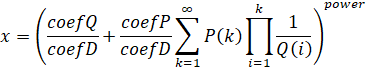Basic Restrictions:

• "Power" is either 1 or -1. If omitted, it defaults to 1.

• "CoefficientP" is a non-zero word-sized signed integer:
• 32-bit Binary: Coefficient ∈ [-231, 231)
• 64-bit Binary: Coefficient ∈ [-263, 263)

• "CoefficientQ" is a word-sized signed integer:
• 32-bit Binary: CoefficientQ ∈ [-231, 231)
• 64-bit Binary: CoefficientQ ∈ [-263, 263)

• "CoefficientD" is a non-zero word-sized unsigned integer:
• 32-bit Binary: x ∈ [1, 232)
• 64-bit Binary: x ∈ [1, 263)

Polynomial Restrictions:

1. P(x) and Q(x) are non-zero polynomials.
2. Q(x) must be degree 1 or higher.
3. Q(x) is of degree no higher than 62.
4. The coefficients of both polynomials are signed 64-bit integers: [-263, 263)
5. The coefficient of the highest degree is non-zero for both polynomials. (no leading zeros)
6. Q(x) is non-zero for all positive integers.

Tips:

• The series can be made alternating by negating the Q(x) polynomial.
• The series above starts at 1 instead of 0. The coefQ/coefD term is there to let you pull out the first term of a series to fit the formula.
• Coefficients of 0, 1, and -1 for coefP, coefQ, or coefD are recognized by the program and are computationally elided.
• If |coefP| == |coefQ|, the redundant multiply will be factored out.

Performance Metrics:

 Metric Complexity Computational Cost O(n log(n)2) Auxiliary Memory O(n)

Notes:

• This series type is named "Hyperdescent" because it converges extremely quickly. The "expansion factor" of this series type is asympotically 1.0.
• All series of this type, regardless of the P(x) and Q(x) polynomials, have the same asympotic speed. As the # of digits approaches infinity, the ratio of the speeds between any two series of this type approaches 1. However, if Q(x) has factors of 2, the series will run faster at first and will approach the common limit at a slower rate. This is due to y-cruncher's trailing zero optimizations which will remove factors of 2 and compress them into a floating-point exponent.

### SeriesHypergeometric

```{
SeriesHypergeometric : {
Power : -1        CoefficientP : 1        CoefficientQ : 2        CoefficientD : 2        PolynomialP : [0 0 0 2 3]        PolynomialQ : [-1 -6 -12 -8]        PolynomialR : [0 0 0 1]    }
}```

This provides access to y-cruncher's CommonP2B3 Binary Splitting framework. This is a generic series type that allows summation of any hypergeometric series of rationals - subject to restrictions such as convergence and implementation limits.

Computes:

Given:

• coefP = CoefficientP
• coefQ = CoefficientQ
• coefD = CoefficientD
• P(x) = PolynomialP
• Q(x) = PolynomialQ
• R(x) = PolynomialR

The polynomials are arrays of the coefficients in increasing order of degree. The 1st element is the constant.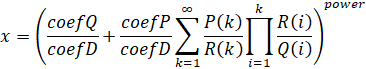Admittedly, this formula may be inconvenient since it does not match the conventional definition of the hypergeometric function. But it was chosen because it matches the implementation. So it is close to metal and any optimizations that are done to the formula will mirror the actual performance improvements. This representation is also slightly more general in that you can use irreducible polynomials that will not factor into a normal rational hypergeometric function.

Basic Restrictions:

• "Power" is either 1 or -1. If omitted, it defaults to 1.

• "CoefficientP" is a non-zero word-sized signed integer:
• 32-bit Binary: Coefficient ∈ [-231, 231)
• 64-bit Binary: Coefficient ∈ [-263, 263)

• "CoefficientQ" is a word-sized signed integer:
• 32-bit Binary: CoefficientQ ∈ [-231, 231)
• 64-bit Binary: CoefficientQ ∈ [-263, 263)

• "CoefficientD" is a non-zero word-sized unsigned integer:
• 32-bit Binary: x ∈ [1, 232)
• 64-bit Binary: x ∈ [1, 263)

Polynomial Restrictions:

1. P(x), Q(x), and R(x) are non-zero polynomials.
2. Q(x) must be degree 1 or higher.
3. Q(x) and R(x) are of degree no higher than 62.
4. The coefficients of all polynomials are signed 64-bit integers: [-263, 263)
5. The coefficient of the highest degree is non-zero for all 3 polynomials. (no leading zeros)
6. Q(x) and R(x) are non-zero for all positive integers.
7. |R(x) / Q(x)| < 0.75 for all x >= 1.

The last restriction enforces a minimum rate of convergence on the series. This restriction is rather broad as it will exclude a large number of valid and convergent series. But unfortunately, it is needed for y-cruncher to work correctly.

For example, y-cruncher will reject the following convergent series: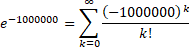This series diverges for the first 1,000,000 terms before it starts to converge. Furthermore, it exhibits destructive cancellation. This is quite typical of Confluent Hypergeometric series for large inputs. But as of version 0.7.7, y-cruncher cannot handle them and will reject all of such series.

Tips:

• The series can be made alternating by negating either the Q(x) or R(x) polynomials. Which you choose will affect the parity.
• The series above starts at 1 instead of 0. The coefQ/coefD term is there to let you pull out the first term of a series to fit the formula.
• Common factors between P(x), Q(x), and R(x) can be removed to make the series faster.
• If R(x) is 1 or -1, use SeriesHyperdescent instead.
• If Q(x) is a power-of-two multiple of R(x), use SeriesBinaryBBP instead.
• Coefficients of 0, 1, and -1 for coefP, coefQ, or coefD are recognized by the program and are computationally elided.
• If |coefP| == |coefQ|, the redundant multiply will be factored out.

Performance Metrics:

 Metric R(x) and Q(x) are same degree R(x) is lower degree than Q(x) R(x) is higher degree than Q(x) Computational Cost O(n log(n)3) O(n log(n)2) Series is Divergent Auxiliary Memory O(n) O(n)

### SeriesBinaryBBP

```{
SeriesBinaryBBP : {
Power : 1        CoefficientP : 1        CoefficientQ : 0        CoefficientD : 1
Alternating : "true"
PowerCoef : -10
PowerShift : 9        PolynomialP :         PolynomialQ : [-3 4]    }
}```

This provides access to y-cruncher's BinaryBBP Binary Splitting framework. This is an optimized special case of SeriesHypergeometric where Q(x) is a power-of-two multiple of R(x). All BBP-type formula with a base 2 radix will fall into this series type.

Computes:

Given:

• coefP = CoefficientP
• coefQ = CoefficientQ
• coefD = CoefficientD
• r = PowerCoef
• s = PowerShift
• P(x) = PolynomialP
• Q(x) = PolynomialQ

The polynomials are arrays of the coefficients in increasing order of degree. The 1st element is the constant.

Computes one of the following depending on whether "Alternating" is true: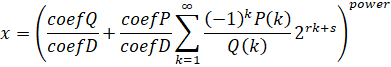As with SeriesHypergeometric, this formula is slightly unconventional.

Basic Restrictions:

• "Power" is either 1 or -1. If omitted, it defaults to 1.

• "CoefficientP" is a non-zero word-sized signed integer:
• 32-bit Binary: Coefficient ∈ [-231, 231)
• 64-bit Binary: Coefficient ∈ [-263, 263)

• "CoefficientQ" is a word-sized signed integer:
• 32-bit Binary: CoefficientQ ∈ [-231, 231)
• 64-bit Binary: CoefficientQ ∈ [-263, 263)

• "CoefficientD" is a non-zero word-sized unsigned integer:
• 32-bit Binary: x ∈ [1, 232)
• 64-bit Binary: x ∈ [1, 263)

• "Alternating" is either "true" or "false".
• "PowerCoef" is a negative integer ∈ [-1024, -1]
• "PowerShift" is a small integer ∈ [-1023, 1023]

Polynomial Restrictions:

1. P(x) and Q(x) are non-zero polynomials.
2. Q(x) must be degree 1 or higher.
3. Q(x) is of degree no higher than 62.
4. The coefficients of both polynomials are signed 64-bit integers: [-263, 263)
5. The coefficient of the highest degree is non-zero for both polynomials. (no leading zeros)
6. Q(x) is non-zero for all positive integers.

Unlike the generic SeriesHypergeometric, the BinaryBBP series has no issues with irregular convergence or destructive cancellation.

Tips:

• The series above starts at 1 instead of 0. The coefQ/coefD term is there to let you pull out the first term of a series to fit the formula. Though in most cases for BBP-type formula, a simple index shift is sufficient and coefQ can be set to zero.
• Common factors between P(x) and Q(x) can be removed to make the series faster.
• Coefficients of 0, 1, and -1 for coefP, coefQ, or coefD are recognized by the program and are computationally elided.
• If |coefP| == |coefQ|, the redundant multiply will be factored out.

Performance Metrics:

 Metric Complexity Computational Cost O(n log(n)3) Auxiliary Memory O(n)

Function Templates:

These are not built-in functions, but are templates for various functions.

### ArcTan(1/x) - Taylor Series

These are useful for building Machin-like ArcTan() formulas for Pi.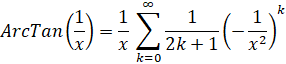Template using SeriesHypergeometric: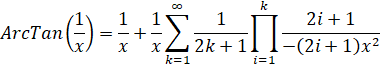coefP = 1

coefQ = 1

coefD = x

P(k) = 1

Q(k) = -(2k+1) x2

R(k) = 2k+1

```{
SeriesHypergeometric : {        CoefficientP : 1        CoefficientQ : 1        CoefficientD : x        PolynomialP :         PolynomialQ : [-x2 -2x2]        PolynomialR : [1 2]    }
}```

Unlike ArcCoth(x), y-cruncher has no native implementation of ArcTan(). It's not needed since nothing to date can touch Chudnovsky and Ramanujan's formulas.

### ArcTan(1/x) - Euler Series

Euler's series for ArcTan() is slightly faster than the Taylor series.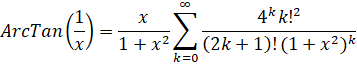Template using SeriesHypergeometric: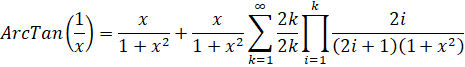coefP = x

coefQ = x

coefD = 1+x2

P(k) = 2k

Q(k) = (2k+1)(1+x2)

R(k) = 2k

If x is an odd number, a factor of 2 can be removed from P(k), Q(k), and R(k).

```{
SeriesHypergeometric : {        CoefficientP : x        CoefficientQ : x        CoefficientD : 1+x2        PolynomialP : [0 2]        PolynomialQ : [-1-x2 -2-2x2]        PolynomialR : [0 2]    }
}```

See Pi - Machin.cfg for an example.

### ArcTanh(x) - Inverse Hyperbolic Tangent

The following computes ArcTanh(x) for any real x ∈ (-1, 1):

`{    Shift : [        {Log : {Scope : {            Locals : {                arg : {...}     //  <-- Argument goes here.            }            Formula : {                Divide : [                    {LinearCombination : [[1 "arg"][1 1]]}                    {LinearCombination : [[-1 "arg"][1 1]]}                ]            }        }}}        -1    ]}`

If you need to reference Pi or log(2) in your argument, the following version is more efficient.

`{    Shift : [        {Scope : {            Locals : {                pi : {Pi : {}}                log2 : {Log : 2}                arg : {...}     //  <-- Argument goes here. You can reference "pi" and "log2".            }            Formula : {                Log-AGM : {                    Pi : "pi"                    Log2 : "log2"                    Argument : {                        Divide : [                            {LinearCombination : [[1 "arg"][1 1]]}                            {LinearCombination : [[-1 "arg"][1 1]]}                        ]                    }                }            }        }}        -1    ]}`

### ArcCoth(x) - Inverse Hyperbolic Cotangent

The following computes ArcCoth(x) for any real x ∈ (-∞, -1) or (1, ∞):

`{    Shift : [        {Log : {Scope : {            Locals : {                arg : {...}     //  <-- Argument goes here.            }            Formula : {                Divide : [                    {LinearCombination : [[1 "arg"][1 1]]}                    {LinearCombination : [[1 "arg"][1 -1]]}                ]            }        }}}        -1    ]}`

If you need to reference Pi or log(2) in your argument, the following version is more efficient.

`{    Shift : [        {Scope : {            Locals : {                pi : {Pi : {}}                log2 : {Log : 2}                arg : {...}     //  <-- Argument goes here. You can reference "pi" and "log2".            }            Formula : {                Log-AGM : {                    Pi : "pi"                    Log2 : "log2"                    Argument : {                        Divide : [                            {LinearCombination : [[1 "arg"][1 1]]}                            {LinearCombination : [[1 "arg"][1 -1]]}                        ]                    }                }            }        }}        -1    ]}`

### ArcSinh(x) - Inverse Hyperbolic Sine

The following computes ArcSinh(x) for any real x:

`{    Log : {Scope : {        Locals : {            arg : {...}     //  <-- Argument goes here.        }        Formula : {            LinearCombination : [                [1 "arg"]                [1 {Sqrt : {                    LinearCombination : [                        [1 {Power : ["arg" 2]}]                        [1 1]                    ]                }}]            ]        }    }}}`

If you need to reference Pi or log(2) in your argument, the following version is more efficient.

`{    Scope : {        Locals : {            pi : {Pi : {}}            log2 : {Log : 2}            arg : {...}     //  <-- Argument goes here. You can reference "pi" and "log2".        }        Formula : {            Log-AGM : {                Pi : "pi"                Log2 : "log2"                Argument : {                    LinearCombination : [                        [1 "arg"]                        [1 {Sqrt : {                            LinearCombination : [                                [1 {Power : ["arg" 2]}]                                [1 1]                            ]                        }}]                    ]                }            }        }    }}`

### ArcCosh(x) - Inverse Hyperbolic Cosine

The following computes ArcCosh(x) for any real x:

`{    Log : {Scope : {        Locals : {            arg : {...}     //  <-- Argument goes here.        }        Formula : {            LinearCombination : [                [1 "arg"]                [1 {Sqrt : {                    LinearCombination : [                        [1 {Power : ["arg" 2]}]                        [1 -1]                    ]                }}]            ]        }    }}}`

If you need to reference Pi or log(2) in your argument, the following version is more efficient.

`{    Scope : {        Locals : {            pi : {Pi : {}}            log2 : {Log : 2}            arg : {...}     //  <-- Argument goes here. You can reference "pi" and "log2".        }        Formula : {            Log-AGM : {                Pi : "pi"                Log2 : "log2"                Argument : {                    LinearCombination : [                        [1 "arg"]                        [1 {Sqrt : {                            LinearCombination : [                                [1 {Power : ["arg" 2]}]                                [1 -1]                            ]                        }}]                    ]                }            }        }    }}`